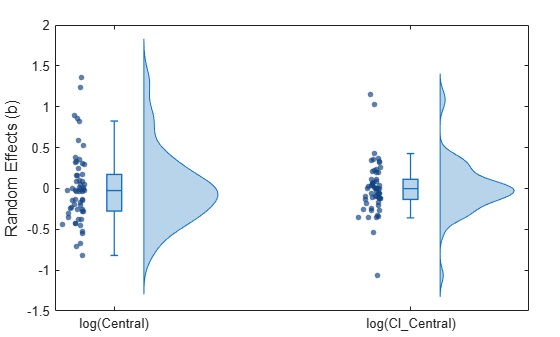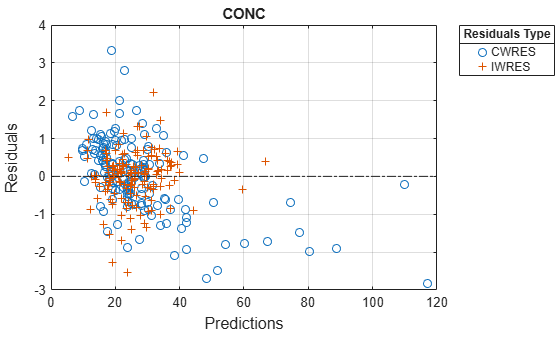# NLMEResults

Results object containing estimation results from nonlinear mixed-effects modeling

## Description

The `NLMEResults` object contains estimation results from fitting a nonlinear mixed-effects model using `sbiofitmixed`.

## Creation

Use the `sbiofitmixed` function to create an `NLMEResults` object.

## Properties

expand all

Table of the estimated fixed effects and their standard errors, specified as a table.

Table of the estimated random effects for each group, specified as a table.

Table of estimated parameter values, including fixed and random effects, specified as a table.

Table of estimated parameter values, including only fixed effects, specified as a table.

Table of the covariance matrix of the random effects, specified as a table.

Statistics returned by the `nlmefit` (Statistics and Machine Learning Toolbox) and `nlmefitsa` (Statistics and Machine Learning Toolbox) algorithm, specified as a structure array.

Covariate names, specified as a cell array of character vectors.

Data used for fitting, specified as a `groupedData` object.

This `Data` property contains a copy of `groupedData` specified as the input data in the `sbiofitmixed` call or the Data property of a `fitproblem` object.

Estimated parameter names, specified as a cell array of character vectors.

Table describing the error models and estimated error model parameters, specified as a table.

The table has one row with three variables: `ErrorModel`, `a`, and `b`. The `ErrorModel` variable is categorical. The variables `a` and `b` can be `NaN` when they do not apply to a particular error model.

There are four built-in error models. Each model defines the error using a standard mean-zero and unit-variance (Gaussian) variable e, the function value f, and one or two parameters a and b. In SimBiology®, the function f represents simulation results from a SimBiology model.

• `'constant'`: $y=f+ae$

• `'proportional'`: $y=f+b|f|e$

• `'combined'`: $y=f+\left(a+b|f|\right)e$

• `'exponential'`: $y=f\ast \mathrm{exp}\left(ae\right)$

Name of the estimation function, specified as `'nlmefit'` or `'nlmefitsa'`.

Maximized loglikelihood for the fitted model, specified as a scalar.

Akaike Information Criterion (AIC), specified as a scalar. The AIC is calculated as `AIC = 2*(-LogLikelihood + P)`, where P is the number of parameters. For details, see `nlmefit` (Statistics and Machine Learning Toolbox).

Bayes Information Criterion (BIC), specified as a scalar. The BIC is calculated as `BIC = -2*LogLikelihood + P*log(N)`, where N is the number of observations or groups, and P is the number of parameters. For details, see `nlmefit` (Statistics and Machine Learning Toolbox).

Degrees of freedom for error (DFE), specified as a scalar. The DFE is calculated as `DFE = N-P`, where N is the number of observations and P is the number of parameters.

Note

If you are using the `nlmefitsa` method, `Loglikelihood`, `AIC`, and `BIC` properties are empty by default. To calculate these values, specify the `'LogLikMethod'` option of `nlmefitsa` (Statistics and Machine Learning Toolbox) when you run `sbiofitmixed` as follows.

```opt.LogLikMethod = 'is'; fitResults = sbiofitmixed(...,'nlmefitsa',opt);```

## Object Functions

 `boxplot` Create box plot showing the variation of estimated SimBiology model parameters `covariateModel` Return a copy of the covariate model that was used for the nonlinear mixed-effects estimation using `sbiofitmixed` `fitted` Return the simulation results of a fitted nonlinear mixed-effects model `plot` Compare simulation results to the training data, creating a time-course subplot for each group `plotActualVersusPredicted` Compare predictions to actual data, creating a subplot for each response `plotResidualDistribution` Plot the distribution of the residuals `plotResiduals` Plot the residuals for each response, using the time, group, or prediction as the x-axis `predict` Simulate and evaluate fitted SimBiology model `random` Simulate a SimBiology model, adding variations by sampling the error model

## Examples

collapse all

This example uses data collected on 59 preterm infants given phenobarbital during the first 16 days after birth . Each infant received an initial dose followed by one or more sustaining doses by intravenous bolus administration. A total of between 1 and 6 concentration measurements were obtained from each infant at times other than dose times, for a total of 155 measurements. Infant weights and APGAR scores (a measure of newborn health) were also recorded.

`load pheno.mat ds`

Convert the dataset to a `groupedData object`, a container for holding tabular data that is divided into groups. It can automatically identify commonly used variable names as the grouping variable or independent (time) variable. Display the properties of the data and confirm that `GroupVariableName` and `IndependentVariableName` are correctly identified as `'ID'` and `'TIME'`, respectively.

```data = groupedData(ds); data.Properties```
```ans = struct with fields: Description: '' UserData: [] DimensionNames: {'Observations' 'Variables'} VariableNames: {'ID' 'TIME' 'DOSE' 'WEIGHT' 'APGAR' 'CONC'} VariableDescriptions: {} VariableUnits: {} VariableContinuity: [] RowNames: {} CustomProperties: [1x1 matlab.tabular.CustomProperties] GroupVariableName: 'ID' IndependentVariableName: 'TIME' ```

Create a simple one-compartment PK model with bolus dosing and linear clearance to fit such data. Use the `PKModelDesign` object to construct the model. Each compartment is defined by a name, dosing type, a clearance type, and whether or not the dosing requires a lag parameter. After constructing the model, you can also get a `PKModelMap` object `map` that lists the names of species and parameters in the model that are most relevant for fitting.

```pkmd = PKModelDesign; addCompartment(pkmd,'Central','DosingType','Bolus',... 'EliminationType','linear-clearance',... 'HasResponseVariable',true,'HasLag',false); [onecomp, map] = pkmd.construct;```

Describe the experimentally measured response by mapping the appropriate model component to the response variable. In other words, indicate which species in the model corresponds to which response variable in the data. The `PKModelMap` property `Observed` indicates that the relevant species in the model is `Drug_Central`, which represents the drug concentration in the system. The relevant data variable is `CONC`, which you visualized previously.

`map.Observed`
```ans = 1x1 cell array {'Drug_Central'} ```

Map the `Drug_Central` species to the `CONC` variable.

`responseMap = 'Drug_Central = CONC';`

The parameters to estimate in this model are the volume of the central compartment `Central` and the clearance rate `Cl_Central`. The `PKModelMap` property `Estimated` lists these relevant parameters. The underlying algorithm of `sbiofit` assumes parameters are normally distributed, but this assumption may not be true for biological parameters that are constrained to be positive, such as volume and clearance. Specify a log transform for the estimated parameters so that the transformed parameters follow a normal distribution. Use an `estimatedInfo` object to define such transforms and initial values (optional).

`map.Estimated`
```ans = 2x1 cell {'Central' } {'Cl_Central'} ```

Define such estimated parameters, appropriate transformations, and initial values.

`estimatedParams = estimatedInfo({'log(Central)','log(Cl_Central)'},'InitialValue',[1 1]);`

Each infant received a different schedule of dosing. The amount of drug is listed in the data variable `DOSE`. To specify these dosing during fitting, create dose objects from the data. These objects use the property `TargetName` to specify which species in the model receives the dose. In this example, the target species is `Drug_Central`, as listed by the `PKModelMap` property `Dosed`.

`map.Dosed`
```ans = 1x1 cell array {'Drug_Central'} ```

Create a sample dose with this target name and then use the `createDoses` method of `groupedData` object `data` to generate doses for each infant based on the dosing data `DOSE`.

```sampleDose = sbiodose('sample','TargetName','Drug_Central'); doses = createDoses(data,'DOSE','',sampleDose);```

Fit the model.

`[nlmeResults,simI,simP] = sbiofitmixed(onecomp,data,responseMap,estimatedParams,doses,'nlmefit');`

Visualize the fitted results using individual-specific parameter estimates.

`plot(nlmeResults,'ParameterType','individual');`Visualize the fitted results using population parameter estimates.

`plot(nlmeResults,'ParameterType','population');`Display the variation of estimated parameters using boxplot.

`boxplot(nlmeResults)`Compare the model predictions to the actual data.

`plotActualVersusPredicted(nlmeResults)`Plot the distribution of residuals.

`plotResidualDistribution(nlmeResults)`Plot residuals for each response using the model predictions on x-axis.

`plotResiduals(nlmeResults,'Predictions')`## Version History

Introduced in R2014a